# 波动方程的高阶间断有限元方法High Order Discontinuous Finite Element Method for Wave Equation

DOI: 10.12677/PM.2021.114081, PDF, HTML, XML, 下载: 23  浏览: 58  科研立项经费支持

Abstract: In recent years, discontinuous finite element method has been widely used in solving hyperbolic equations. Compared with traditional finite element method and difference method, discontinuous finite element method has many advantages. In this paper, the discontinuous finite element analysis is applied to the hyperbolic wave equation. By constructing the strong form of the discon-tinuous finite element and selecting the appropriate numerical flux, the smaller error and higher convergence order are obtained compared with the exact solution, and the theoretical convergence order of the discontinuous finite element is reached.

1. 引言

2. 波动方程及其间断有限元方法

2.1. 波动方程

$\left\{\begin{array}{l}\frac{{\partial }^{2}U\left(x,t\right)}{\partial {t}^{2}}-{c}^{2}\frac{{\partial }^{2}U\left(x,t\right)}{\partial {x}^{2}}=0\text{}x\in \left[L,R\right],\text{}t>0\\ U\left(x,0\right)=f\left(x\right)\text{}x\in \left[L,R\right]\\ \frac{\partial U\left(x,0\right)}{\partial t}=g\left(x\right)\text{}x\in \left[L,R\right]\end{array}\text{}$

$c\frac{\partial U}{\partial x}=\frac{\partial P}{\partial t}.$

$\left\{\begin{array}{l}\frac{{\partial }^{2}U}{\partial {t}^{2}}-c\frac{\partial }{\partial x}\left(\frac{\partial P}{\partial t}\right)=0\\ c\frac{\partial U}{\partial x}=\frac{\partial P}{\partial t}\end{array}\text{}⇒\text{}\left\{\begin{array}{l}\frac{\partial U}{\partial t}-c\frac{\partial P}{\partial x}=0\\ \frac{\partial P}{\partial t}\text{=}c\frac{\partial U}{\partial x}\end{array}\text{}\text{.}$

$W=\left[\begin{array}{l}U\\ P\end{array}\right]\text{ }V=\left[\begin{array}{cc}0& -c\\ -c& 0\end{array}\right]\text{ }$

2.2. 空间域间断有限元方法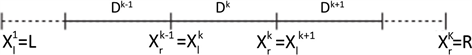Figure 1. Element division of calculation area

$x\in {D}^{k}:x\left(r\right)={x}_{l}^{k}+\frac{1+r}{2}{h}^{k},\text{ }{h}^{k}={x}_{r}^{k}-{x}_{l}^{k}.$

${\int }_{{D}^{k}}{R}_{h}\left(x,t\right){\phi }_{n}\left(x\right)\text{d}x=0,\text{ }1\le n\le {N}_{p}.$

${\int }_{{\text{D}}^{k}}\left(\frac{\partial {W}_{h}^{k}}{\partial t}{\phi }_{n}-V{W}_{h}^{k}\frac{\text{d}{\phi }_{n}}{\text{d}x}\right)\text{d}x=-{\left[V{W}_{h}^{k}{\phi }_{n}\right]}_{{x}_{i}^{k}}^{{x}_{r}^{k}}=-{\int }_{\partial {D}^{k}}\stackrel{^}{n}\cdot V{W}_{h}^{k}{\phi }_{n}\text{d}x,\text{}1\le n\le {N}_{p}.$

${\int }_{{D}^{k}}{\mathcal{R}}_{h}\left(x,t\right){\phi }_{n}\text{d}x={\int }_{\partial {D}^{k}}\stackrel{^}{n}\cdot \left(V{W}_{h}^{k}-{\left(V{W}_{h}\right)}^{*}\right){\phi }_{n}\text{d}x,\text{}1\le n\le {N}_{p}.$

${\mathcal{M}}^{k}\frac{\text{d}{W}_{h}^{k}}{\text{d}t}+V\cdot \mathcal{S}{W}_{h}^{k}={\left[{\mathcal{l}}^{k}\left(x\right)\left(V{W}_{h}^{k}-{\left(VW\right)}^{*}\right)\right]}_{{x}_{l}^{k}}^{{x}_{r}^{k}}.$

$\begin{array}{l}{\mathcal{M}}_{ij}^{k}={\int }_{{x}_{l}^{k}}^{{x}_{r}^{k}}{\phi }_{i}^{k}\left(x\right){\phi }_{j}^{k}\left(x\right)\text{d}x=\frac{{h}^{k}}{2}{\int }_{-1}^{1}{\phi }_{i}\left(r\right){\phi }_{j}\left(r\right)\text{d}r\\ {\mathcal{S}}_{ij}^{k}={\int }_{{x}_{l}^{k}}^{{x}_{r}^{k}}{\phi }_{i}^{k}\left(x\right)\frac{\text{d}{\phi }_{j}^{k}\left(x\right)}{\text{d}x}\text{d}x={\int }_{-1}^{1}{\phi }_{i}\left(r\right)\frac{\text{d}{\phi }_{j}\left(r\right)}{\text{d}r}\text{d}r\end{array}$ .

$\frac{\text{d}{U}_{h}^{k}}{\text{d}t}+\frac{-c}{{J}^{k}}{\mathcal{D}}_{r}{P}_{h}^{k}=\frac{1}{{J}^{k}}{\mathcal{M}}^{-1}{\left[{\phi }^{k}\left(x\right)\left(\left(-c\right){H}_{h}^{k}-\left(-c\right){H}^{*}\right)\right]}_{{x}_{l}^{k}}^{{x}_{r}^{k}}=\frac{1}{{J}^{k}}{\mathcal{M}}^{-1}{\oint }_{{x}_{l}^{k}}^{{x}_{r}^{k}}\stackrel{^}{n}\cdot \left(\left(-c\right){H}_{h}^{k}-\left(-c\right){H}^{*}\right){\phi }^{k}\left(x\right)\text{d}x.$

$\frac{\text{d}{P}_{h}^{k}}{\text{d}t}+\frac{-c}{{J}^{k}}{\mathcal{D}}_{r}{U}_{h}^{k}=\frac{1}{{J}^{k}}{\mathcal{M}}^{-1}{\left[{\phi }^{k}\left(x\right)\left(\left(-c\right){E}_{h}^{k}-\left(-c\right){E}^{*}\right)\right]}_{{x}_{l}^{k}}^{{x}_{r}^{k}}=\frac{1}{{J}^{k}}{\mathcal{M}}^{-1}{\oint }_{{x}_{l}^{k}}^{{x}_{r}^{k}}\stackrel{^}{n}\cdot \left(\left(-c\right){E}_{h}^{k}-\left(-c\right){E}^{*}\right){\phi }^{k}\left(x\right)\text{d}x.$

${\left(\mathcal{M}{\mathcal{D}}_{r}\right)}_{\left(i,j\right)}=\underset{n=1}{\overset{{N}_{p}}{\sum }}{\mathcal{M}}_{in}{\mathcal{D}}_{r,\left(n,j\right)}={\underset{n=1}{\overset{{N}_{p}}{\sum }}{\int }_{-1}^{1}{\phi }_{i}\left(r\right){\phi }_{n}\left(r\right)\frac{\text{d}{\phi }_{j}}{\text{d}r}|}_{{r}_{n}}\text{d}r={{\int }_{-1}^{1}{\phi }_{i}\left(r\right)\underset{n=1}{\overset{{N}_{p}}{\sum }}\frac{\text{d}{\phi }_{j}}{\text{d}r}|}_{{r}_{n}}{\phi }_{n}\left(r\right)\text{d}r={\int }_{-1}^{1}{\phi }_{i}\left(r\right)\frac{\text{d}{\phi }_{j}\left(r\right)}{\text{d}r}\text{d}r={\mathcal{S}}_{ij}.$

2.3. 时间离散

$\frac{\text{d}{W}_{h}}{\text{d}t}={\mathcal{L}}_{h}\left({W}_{h},t\right).$

$\left\{\begin{array}{l}{u}^{\left(0\right)}={W}^{\left(n\right)}\\ {u}^{\left(i\right)}=\underset{l=0}{\overset{i-1}{\sum }}\left[{\alpha }_{il}{u}^{\left(l\right)}+{\beta }_{il}\Delta tL\left({u}^{\left(l\right)}\right)\right]\text{}i=1,\cdots ,r\\ {W}^{\left(n+1\right)}={u}^{\left( r \right)}\end{array}$

$\begin{array}{l}{p}^{\left(0\right)}={W}^{n}\\ \left\{\begin{array}{l}{k}^{\left(i\right)}={a}_{i}{k}^{\left(i-1\right)}+\Delta t{\mathcal{L}}_{h}\left({p}^{\left(i-1\right)},{t}^{n}+{c}_{i}\Delta t\right),\text{}i\in \left[1,\cdots ,5\right]\\ {p}^{\left(i\right)}={p}^{\left(i-1\right)}+{b}_{i}{k}^{\left(i\right)}\end{array}\\ {W}^{n+1}={p}^{\left( 5 \right)}\end{array}$

3. 计算方法的实现

3.1. 基函数

${\mathcal{M}}_{ij}={\left({\phi }_{i},{\phi }_{j}\right)}_{I}\text{=}{\int }_{-1}^{1}{\phi }_{i}{\phi }_{j}\text{d}x\text{=}\frac{1}{i+j-1}\left[1+{\left(-1\right)}^{i+j}\right].$

${\phi }_{n}\left(r\right)={\stackrel{˜}{P}}_{n-1}\left(r\right)=\frac{{P}_{n-1}\left(r\right)}{\sqrt{{\gamma }_{n-1}}}\text{}其中{\gamma }_{n}=\frac{2}{2n+1}.$

3.2. 数值积分

3.3. 数值通量

$\left\{\left\{u\right\}\right\}=\frac{{u}^{-}+{u}^{+}}{2},\text{ }\left[\left[u\right]\right]={\stackrel{^}{n}}^{-}{u}^{-}+{\stackrel{^}{n}}^{+}{u}^{+}.$

${\left(u\right)}^{*}=\left\{\left\{u\right\}\right\}+\frac{1-\alpha }{2}\left[\left[u\right]\right],\text{ }0\le \alpha \le 1$$\alpha =1$ 代表中心通量， $\alpha =0$ 代表迎风通量。

Lax-Friedrichs通量： ${\left(u\right)}^{*}=\left\{\left\{u\right\}\right\}+\frac{C}{2}\left({u}^{+}-{u}^{-}\right)$，其中C与计算区域的情况有关。

3.4. 时间步长

4. 数值实验

4.1. 一阶单波方程

$\left\{\begin{array}{l}\frac{\partial u}{\partial t}+2\text{π}\frac{\partial u}{\partial x}=0\text{ }\text{}x\in \left[-2\text{π},2\text{π}\right],t>0\hfill \\ u\left(x,0\right)=\mathrm{cos}\left(-x\right)\text{}x\in \left[-2\text{π},2\text{π}\right]\hfill \\ u\left(-2\text{π},t\right)=\mathrm{cos}\left(2\text{π}t\right)\text{}t\ge 0\hfill \end{array}$ .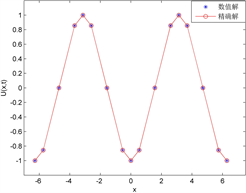(a)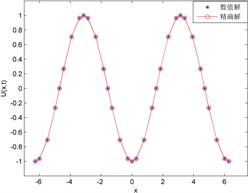(b)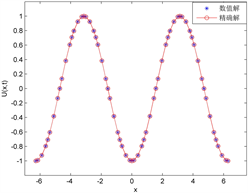(c)

Figure 2. Comparison between numerical solution and exact solution of first-order wave equationTable 1. Error and convergence order of numerical solution

4.2. 二阶波动方程

$\left\{\begin{array}{l}\frac{{\partial }^{2}U\left(x,t\right)}{\partial {t}^{2}}-{c}^{2}\frac{{\partial }^{2}U\left(x,t\right)}{\partial {x}^{2}}=0\text{}x\in \left[-10,10\right],t>0\\ U\left(x,0\right)=\mathrm{sin}\left(x\right)\text{}x\in \left[-10,10\right]\\ \frac{\partial U\left(x,0\right)}{\partial t}=c\mathrm{sin}\left(x\right)\text{}x\in \left[-10,10\right]\end{array}\text{}$ .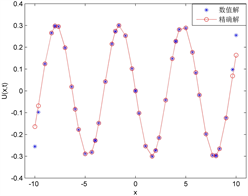(a)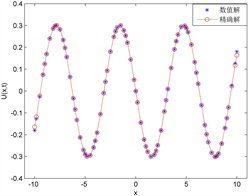(b)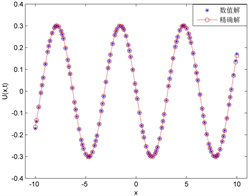(c)

Figure 3. Comparison between numerical solution and exact solution of second-order wave equation

5. 结论

  Reed, W.H. and Hill, T.R. (1973) Triangular Mesh Methods for the Neutron Transport Equation. Los Alamos Report LA-UR-73-479.  Cockburn, B. and Shu, C.W. (1998) The Runge-Kutta Discontinuous Galerkin Method for Con-servation Laws V: Multidimensional Systems. Journal of Computational Physics, 141, 199-224. https://doi.org/10.1006/jcph.1998.5892  Cockburn, B. and Shu, C.W. (1989) TVB Runge-Kutta Local Pro-jection Discontinuous Galerkin Finite Element Method for Conservation Laws II: General Framework. Mathematics of Computation, 52, 411-435. https://doi.org/10.2307/2008474  Hu, F.Q., Hussaini, M.Y. and Rasetarinera, P. (1999) An Analysis of the Discontinuous Galerkin Method for Wave Propagation Problems. Journal of Computational Physics, 151, 921-946. https://doi.org/10.1006/jcph.1999.6227  Cockburn, B. and Shu, C.W. (2001) Runge-Kutta Discontinuous Galerkin Methods for Convection-Dominated Problems. Journal of Scientific Computing, 16, 173-261. https://doi.org/10.1023/A:1012873910884  章晓. 求解波动方程的近似解析指数时间差分方法及其数值模拟[D]: [硕士学位论文]. 北京: 清华大学, 2013.  贺茜君. 求解波动方程的间断有限元方法及其波场模拟[D]: [博士学位论文]. 北京: 清华大学, 2015.  陈二云. 间断有限元数值方法研究及复杂冲击流场的数值模拟[D]: [博士学位论文]. 南京: 南京理工大学, 2008.  陆金甫, 关治. 偏微分方程数值解法[M]. 北京: 清华大学出版社, 2004.  Shu, C.W. (1988) Total-Variation-Diminishing Time Discretizations. SIAM Journal on Scientific and Statistical Computing, 9, 1073-1084. https://doi.org/10.1137/0909073  栾睿琦. 间断有限元地震波场正演模拟[D]: [硕士学位论文] . 北京: 中国石油大学, 2019.  张铁. 间断有限元理论与方法[M]. 北京: 科学出版社, 2012.